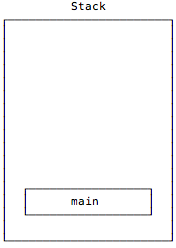# recursion

A Scala ‘foldLeft’ function written using recursion alvin January 20, 2018 - 4:25pm

As a short note, here’s some Scala source code that shows how to write a `foldLeft` function using recursion:

This is a page from my book, Functional Programming, Simplified

## Appendix: Recursion is Great, But ... (Scala’s fold and reduce)

“Folds can be used to implement any function where you traverse a list once, element by element, and then return something based on that. Whenever you want to traverse a list to return something, chances are you want a fold. That’s why folds are, along with maps and filters, one of the most useful types of functions in functional programming.”

From the book, Learn You a Haskell for Great Good!

This is a page from my book, Functional Programming, Simplified

## Scala Recursion Lessons (Section)

This section contains lessons on recursion (recursive programming) in Scala. The lessons in this section are listed below.

This is a page from my book, Functional Programming, Simplified

## Tail-Recursive Algorithms in Scala

“Tail recursion is its own reward.”

From the “Functional” cartoon on xkcd.com.

## Goals

The main goal of this lesson is to solve the problem shown in the previous lessons: Simple recursion creates a series of stack frames, and for algorithms that require deep levels of recursion, this creates a `StackOverflowError` (and crashes your program).

This is a page from my book, Functional Programming, Simplified

## A Visual Look at JVM Stacks and Frames

Given the background information of the previous lesson, let’s take a visual look at how the JVM stack and stack frames work by going back to our recursive sum function from the previous lesson.

Before the `sum` function is initially called, the only thing on the call stack is the application’s `main` method:Then main calls `sum` with `List(1,2,3)`, which I show here without the “List” to keep things simple:

This is a page from my book, Functional Programming, Simplified

## JVM Stacks and Stack Frames

For functions without deep levels of recursion, there’s nothing wrong with the algorithms shown in the previous lessons. I use this simple, basic form of recursion when I know that I’m working with limited data sets. But in applications where you don’t know how much data you might be processing, it’s important that your recursive algorithms are tail-recursive, otherwise you’ll get a nasty `StackOverflowError`.

For instance, if you run the `sum` function from the previous lessons with a larger list, like this:

This is a page from my book, Functional Programming, Simplified

## Recursion: Thinking Recursively

“To understand recursion,
one must first understand recursion.”

Stephen Hawking

## Goal

This lesson has one primary goal: to show that the thought process followed in writing the `sum` function follows a common recursive programming “pattern.” Indeed, when you write recursive functions you’ll generally follow the three-step process shown in this lesson.

This is a page from my book, Functional Programming, Simplified

## Recursion: A Conversation Between Two Developers

As an homage to one of my favorite Lisp books — an early version of what is now The Little Schemer — this lesson shows a little question and answer interaction that you can imagine happening between two Scala programmers.

Given this `sum` function:

``````def sum(list: List[Int]): Int = list match {
case Nil => 0
case x :: xs => x + sum(xs)
}``````

I hope this “conversation” will help drive home some of the points about how recursion works: